# Resources tagged with: Enlargements and scale factors

Filter by: Content type:
Age range:
Challenge level:

### There are 14 results

Broad Topics > Transformations and constructions > Enlargements and scale factors### Sierpinski Triangle

##### Age 16 to 18 Challenge Level:

What is the total area of the triangles remaining in the nth stage of constructing a Sierpinski Triangle? Work out the dimension of this fractal.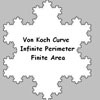### Von Koch Curve

##### Age 16 to 18 Challenge Level:

Make a poster using equilateral triangles with sides 27, 9, 3 and 1 units assembled as stage 3 of the Von Koch fractal. Investigate areas & lengths when you repeat a process infinitely often.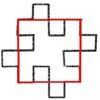### Squareflake

##### Age 16 to 18 Challenge Level:

A finite area inside and infinite skin! You can paint the interior of this fractal with a small tin of paint but you could never get enough paint to paint the edge.##### Age 16 to 18 Challenge Level:

A circle is inscribed in an equilateral triangle. Smaller circles touch it and the sides of the triangle, the process continuing indefinitely. What is the sum of the areas of all the circles?### Golden Triangle

##### Age 16 to 18 Challenge Level:

Three triangles ABC, CBD and ABD (where D is a point on AC) are all isosceles. Find all the angles. Prove that the ratio of AB to BC is equal to the golden ratio.### Conical Bottle

##### Age 14 to 16 Challenge Level:

A right circular cone is filled with liquid to a depth of half its vertical height. The cone is inverted. How high up the vertical height of the cone will the liquid rise?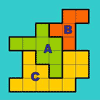### The Rescaled Map

##### Age 14 to 16 Challenge Level:

We use statistics to give ourselves an informed view on a subject of interest. This problem explores how to scale countries on a map to represent characteristics other than land area.### Matter of Scale

##### Age 14 to 16 Challenge Level:

Prove Pythagoras' Theorem using enlargements and scale factors.### Squirty

##### Age 14 to 16 Challenge Level:

Using a ruler, pencil and compasses only, it is possible to construct a square inside any triangle so that all four vertices touch the sides of the triangle.### L-triominoes

##### Age 14 to 16 Challenge Level:

L triominoes can fit together to make larger versions of themselves. Is every size possible to make in this way?### Fit for Photocopying

##### Age 14 to 16 Challenge Level:

Explore the relationships between different paper sizes.### Similar Rectangles

##### Age 14 to 16 Challenge Level:

Can you find the missing length?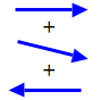### Arrow Arithmetic 1

##### Age 14 to 16 Challenge Level:

The first part of an investigation into how to represent numbers using geometric transformations that ultimately leads us to discover numbers not on the number line.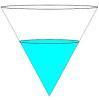### Fill Me up Too

##### Age 14 to 16 Challenge Level:

In Fill Me Up we invited you to sketch graphs as vessels are filled with water. Can you work out the equations of the graphs?